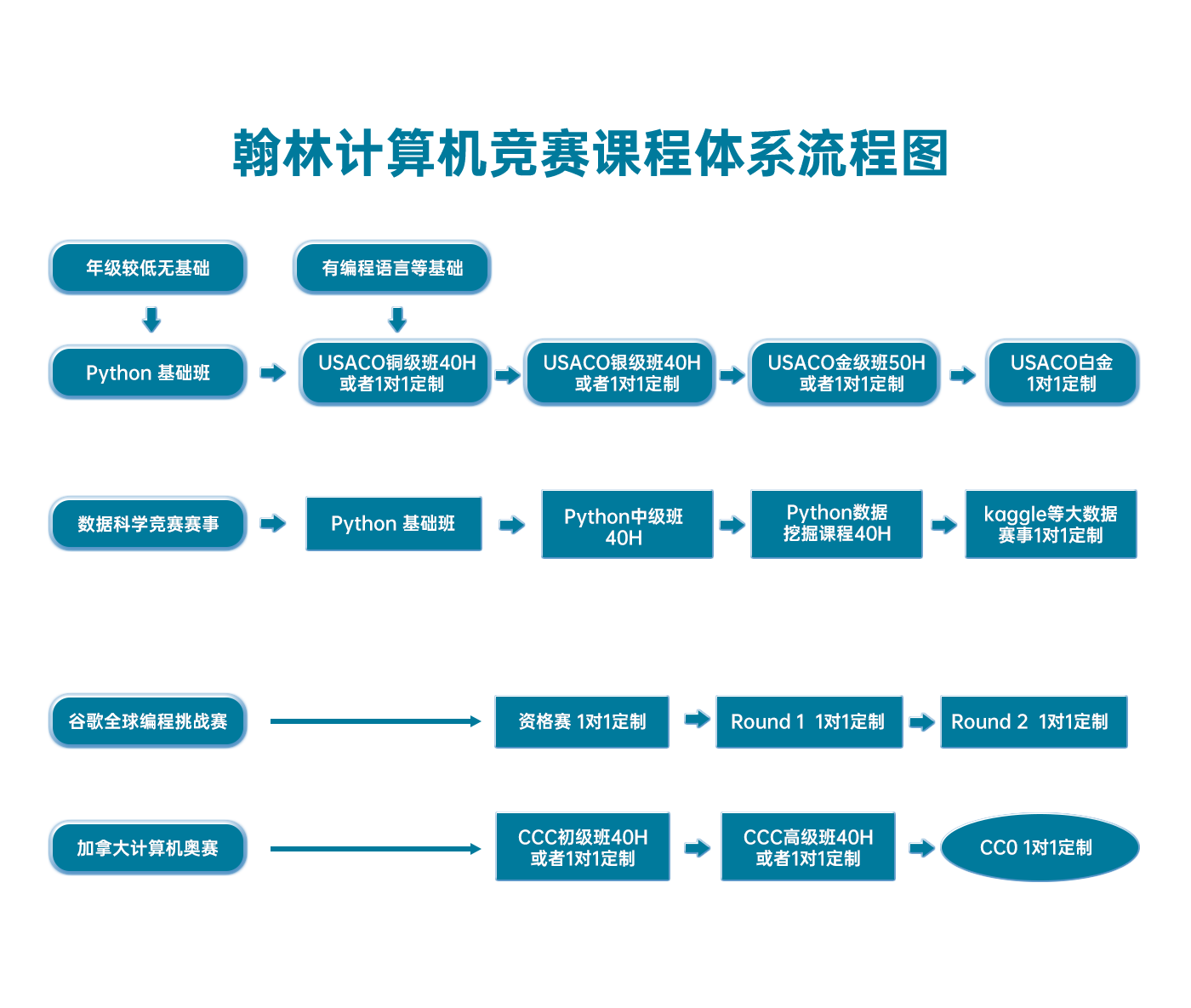# USACO 2015 January Contest, Silver Problem 1. Stampede

USACO2015JAN-S1

(Analysis by Mark Gordon)

The first observation to make in this problem is that we can transform each cow into a time interval corresponding to the interval that the cow is on the line x=0.

Viewed this way the problem can be solved using a sweep line technique. Simply create a sorted list of events corresponding to the times that a cow's interval begins and ends. Every time a cow's interval begins insert it into a set and when it ends remove it from the set. Then to solve the problem we simply need to keep track of which cows in the set ever have the minimum y-coordinate among cows in the set.

See my code for a sample implementation

```#include <iostream>
#include <vector>
#include <algorithm>
#include <set>
#include <cstdio>

using namespace std;

int main() {
freopen("stampede.in", "r", stdin);
freopen("stampede.out", "w", stdout);

int N;
cin >> N;

vector<pair<int, int> > events;
for (int i = 0; i < N; i++) {
int x, y, r;
cin >> x >> y >> r;
x *= -r;

/* We'll use a negative y value to indicate the end of an interval. */
events.push_back(make_pair(x - r, y));
events.push_back(make_pair(x, -y));
}
sort(events.begin(), events.end());

set<int> seen; /* Tracks which intervals we've seen. */
set<int> active; /* Tracks which intervals are currently above us. */
for (int i = 0; i < events.size(); ) {
/* Add/remove any intervals that begin/end at time events[i].first. */
int j;
for (j = i; j < events.size() && events[i].first == events[j].first; j++) {
int y = events[j].second;
if (y > 0) {
active.insert(y);
} else {
active.erase(-y);
}
}

/* Add the first interval we can see to our list of seen intervals. */
if (!active.empty()) {
seen.insert(*active.begin());
}

i = j;
}
cout << seen.size() << endl;

return 0;
}
```

There was also a fairly different approach to this problem that could be coded fairly succinctly using STL's set. We simply store all "interesting" times (times immediate before/during/after an interval starts and ends) in a set and then process the intervals in increasing y values. Using set's lower_bound and upper_bound functions we can find the iterator range representing all times within that interval. If the interval is non-empty we delete everything in the range and increment the number of seen intervals, otherwise the interval is not visible.

```#include <iostream>
#include <vector>
#include <algorithm>
#include <set>
#include <cstdio>

using namespace std;

int main() {
freopen("stampede.in", "r", stdin);
freopen("stampede.out", "w", stdout);

int N;
cin >> N;

set<int> st;
vector<pair<int, pair<int, int> > > A;
for (int i = 0; i < N; i++) {
int x, y, r;
cin >> x >> y >> r;
x *= -r;
A.push_back(make_pair(y, make_pair(x - r, x)));
st.insert(x - r - 1);
st.insert(x - r);
st.insert(x - r + 1);
st.insert(x - 1);
st.insert(x);
st.insert(x + 1);
}
sort(A.begin(), A.end());

int result = 0;
for (int i = 0; i < A.size(); i++) {
auto it = st.lower_bound(A[i].second.first);
auto jt = st.lower_bound(A[i].second.second);
if (it != jt) {
++result;
st.erase(it, jt);
}
}
cout << result << endl;

return 0;
}```

### 翰林USACO课程体系流程图